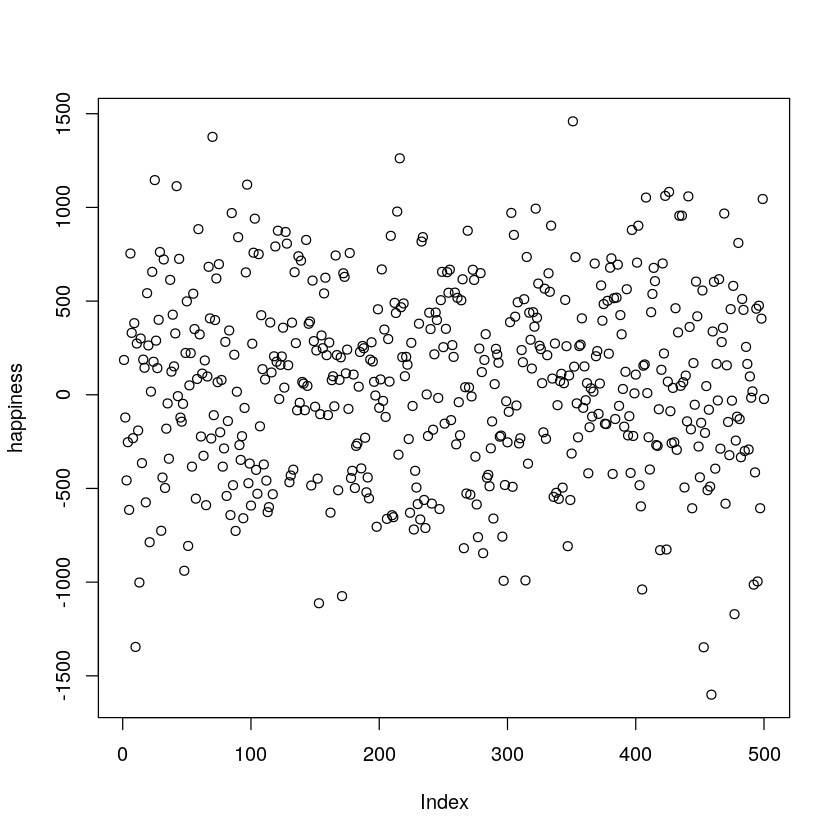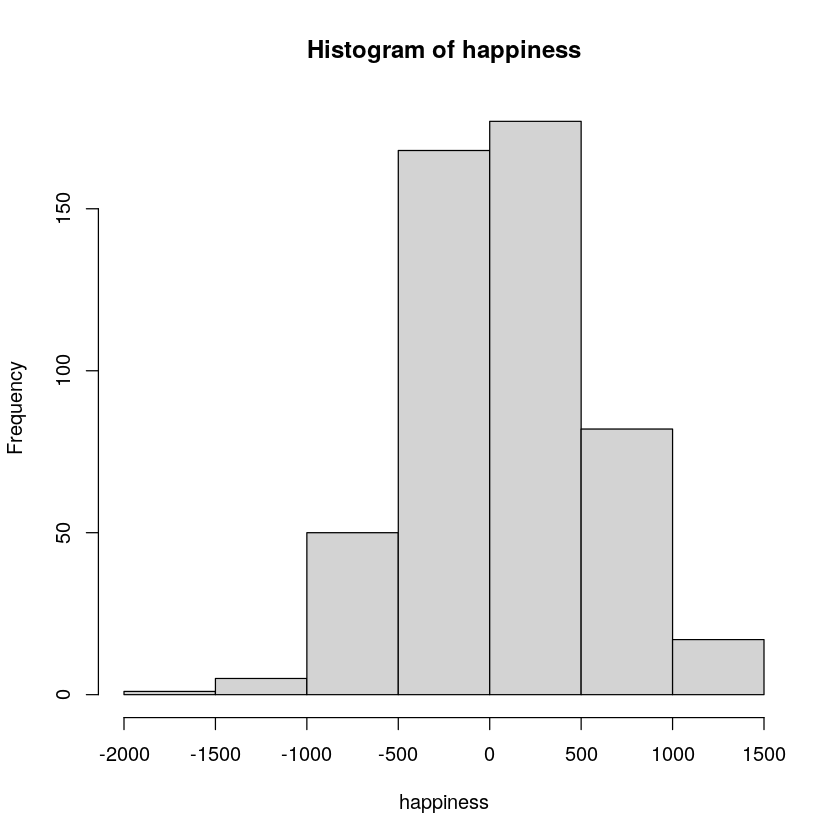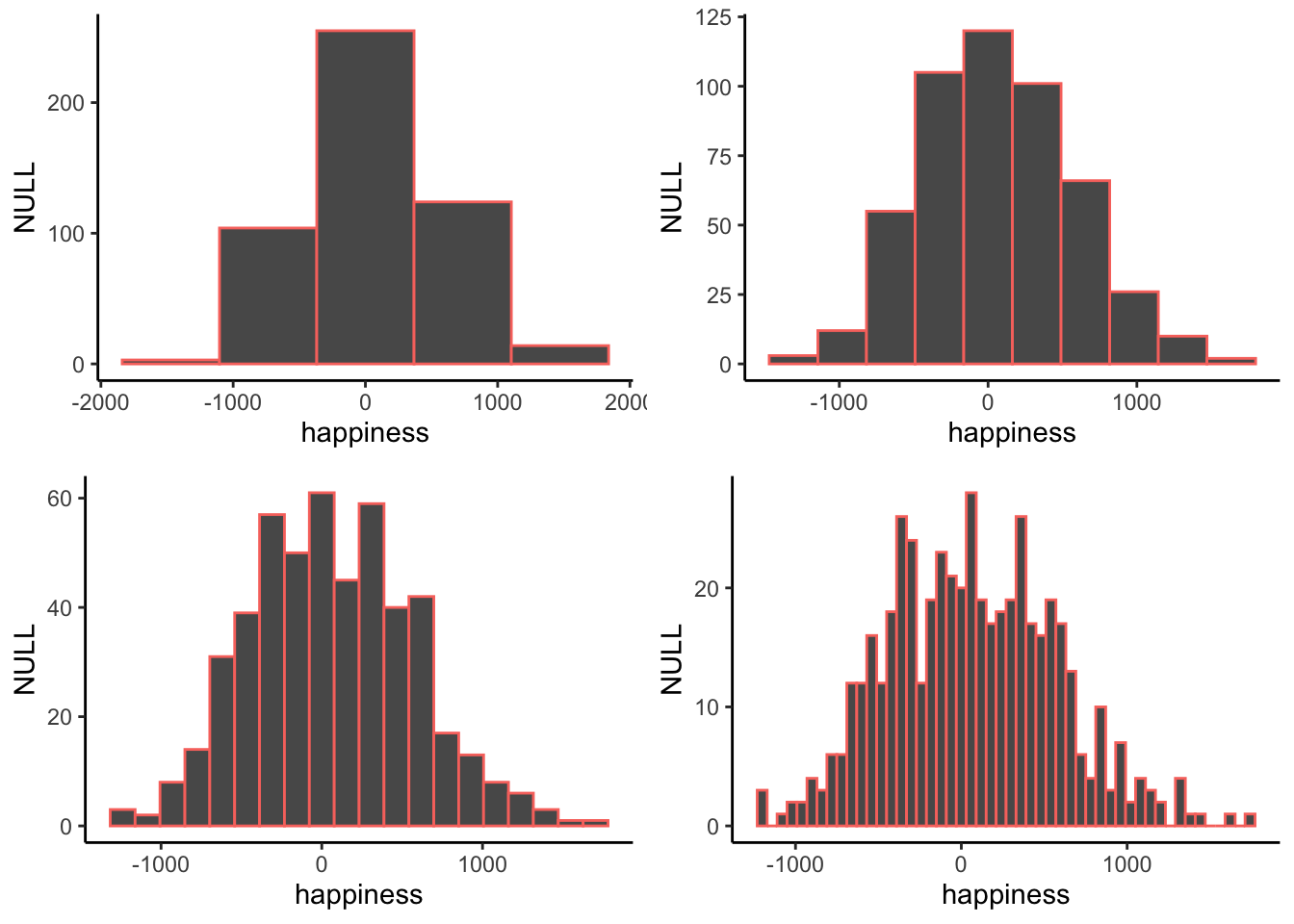# 2.2: Look at the data

$$\newcommand{\vecs}{\overset { \rightharpoonup} {\mathbf{#1}} }$$ $$\newcommand{\vecd}{\overset{-\!-\!\rightharpoonup}{\vphantom{a}\smash {#1}}}$$$$\newcommand{\id}{\mathrm{id}}$$ $$\newcommand{\Span}{\mathrm{span}}$$ $$\newcommand{\kernel}{\mathrm{null}\,}$$ $$\newcommand{\range}{\mathrm{range}\,}$$ $$\newcommand{\RealPart}{\mathrm{Re}}$$ $$\newcommand{\ImaginaryPart}{\mathrm{Im}}$$ $$\newcommand{\Argument}{\mathrm{Arg}}$$ $$\newcommand{\norm}{\| #1 \|}$$ $$\newcommand{\inner}{\langle #1, #2 \rangle}$$ $$\newcommand{\Span}{\mathrm{span}}$$ $$\newcommand{\id}{\mathrm{id}}$$ $$\newcommand{\Span}{\mathrm{span}}$$ $$\newcommand{\kernel}{\mathrm{null}\,}$$ $$\newcommand{\range}{\mathrm{range}\,}$$ $$\newcommand{\RealPart}{\mathrm{Re}}$$ $$\newcommand{\ImaginaryPart}{\mathrm{Im}}$$ $$\newcommand{\Argument}{\mathrm{Arg}}$$ $$\newcommand{\norm}{\| #1 \|}$$ $$\newcommand{\inner}{\langle #1, #2 \rangle}$$ $$\newcommand{\Span}{\mathrm{span}}$$$$\newcommand{\AA}{\unicode[.8,0]{x212B}}$$

We already tried one way of looking at the numbers, and it wasn’t useful. Let’s look at some other ways of looking at the numbers, using graphs.

## Stop, plotting time (o o oh) U can plot this

Let’s turn all of the numbers into dots, then show them in a graph. Note, when we do this, we have not yet summarized anything about the data. Instead, we just look at all of the data in a visual format, rather than looking at the numbers.Figure $$\PageIndex{1}$$: Pretend happiness ratings from 500 people.

Figure $$\PageIndex{1}$$ shows 500 measurements of happiness. The graph has two axes. The horizontal x-axis, going from left to right is labeled “Index”. The vertical y-axis, going up and down, is labelled “happiness”. Each dot represents one measurement of every person’s happiness from our pretend study. Before we talk about what we can and cannot see about the data, it is worth mentioning that the way you plot the data will make some things easier to see and some things harder to see. So, what can we now see about the data?

There are lots of dots everywhere. It looks like there are 500 of them because the index goes to 500. It looks like some dots go as high as 1000-1500 and as low as -1500. It looks like there are more dots in the middle-ish area of the plot, sort of spread about 0.

Take home: we can see all the numbers at once by putting them in a plot, and that is much easier and more helpful than looking at the raw numbers.

OK, so if these dots represent how happy 500 people are, what can we say about those people? First, the dots are kind of all over the place, so different people have different levels of happiness. Are there any trends? Are more people happy than unhappy, or vice-versa? It’s hard to see that in the graph, so let’s make a different one, called a histogram

## Histograms

Making a histogram will be our first act of officially summarizing something about the data. We will no longer look at the individual bits of data, instead we will see how the numbers group together. Let’s look at a histogram of the happiness data, and then explain it.Figure $$\PageIndex{2}$$: A histogram of the happiness ratings.

The dots have disappeared, and now we some bars. Each bar is a summary of the dots, representing the number of dots (frequency count) inside a particular range of happiness, also called bins. For example, how many people gave a happiness rating between 0 and 500? The fifth bar, the one between 0 and 500 on the x-axis, tells you how many. Look how tall that bar is. How tall is it? The height is shown on the y-axis, which provides a frequency count (the number of dots or data points). It looks like around 150 people said their happiness was between 0-500.

More generally, we see there are many bins on the x-axis. We have divided the data into bins of 500. Bin #1 goes from -2000 to -1500, bin #2 goes from -1500 to -1000, and so on until the last bin. To make the histogram, we just count up the number of data points falling inside each bin, then plot those frequency counts as a function of the bins. Voila, a histogram.

What does the histogram help us see about the data? First, we can see the shape of data. The shape of the histogram refers to how it goes up and down. The shape tells us where the data is. For example, when the bars are low we know there isn’t much data there. When the bars are high, we know there is more data there. So, where is most of the data? It looks like it’s mostly in the middle two bins, between -500 and 500. We can also see the range of the data. This tells us the minimums and the maximums of the data. Most of the data is between -1500 and +1500, so no infinite sadness or infinite happiness in our data-set.

When you make a histogram you get to choose how wide each bar will be. For example, below are four different histograms of the very same happiness data. What changes is the width of the bins.Figure $$\PageIndex{3}$$: Four histograms of the same data using different bin widths.

All of the histograms have roughly the same overall shape: From left to right, the bars start off small, then go up, then get small again. In other words, as the numbers get closer to zero, they start to occur more frequently. We see this general trend across all the histograms. But, some aspects of the trend fall apart when the bars get really narrow. For example, although the bars generally get taller when moving from -1000 to 0, there are some exceptions and the bars seem to fluctuate a little bit. When the bars are wider, there are less exceptions to the general trend. How wide or narrow should your histogram be? It’s a Goldilocks question. Make it just right for your data.

This page titled 2.2: Look at the data is shared under a CC BY-SA 4.0 license and was authored, remixed, and/or curated by Matthew J. C. Crump via source content that was edited to the style and standards of the LibreTexts platform; a detailed edit history is available upon request.